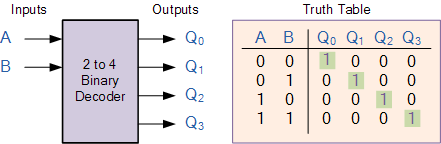# Binary Decoder

## Binary Decoder

Binary DecoderBinary Decoders are another type of digital logic device that has inputs of 2-bit, 3-bit or 4-bit codes depending upon the number of data input lines, so a decoder that has a set of two or more bits will be defined as having an n-bit code, and therefore it will be possible to represent 2n possible values. Thus, a decoder generally decodes a binary value into a non-binary one by setting exactly one of its n outputs to logic “1”.

If a binary decoder receives n inputs (usually grouped as a single Binary or Boolean number) it activates one and only one of its 2n outputs based on that input with all other outputs deactivated.So for example, an inverter ( NOT-gate ) can be classed as a 1-to-2 binary decoder as 1-input and 2-outputs (21) is possible because with an input A it can produce two outputs A and A (not-A) as shown.

Then we can say that a standard combinational logic decoder is an n-to-m decoder, where m ≤ 2n, and whose output, Q is dependent only on its present input states. In other words, a binary decoder looks at its current inputs, determines which binary code or binary number is present at its inputs and selects the appropriate output that corresponds to that binary input.

A Binary Decoder converts coded inputs into coded outputs, where the input and output codes are different and decoders are available to “decode” either a Binary or BCD (8421 code) input pattern to typically a Decimal output code. Commonly available BCD-to-Decimal decoders include the TTL 7442 or the CMOS 4028. Generally a decoders output code normally has more bits than its input code and practical “binary decoder” circuits include, 2-to-4, 3-to-8 and 4-to-16 line configurations.

An example of a 2-to-4 line decoder along with its truth table is given below.

### A 2-to-4 Binary Decoders.This simple example above of a 2-to-4 line binary decoder consists of an array of four AND gates. The 2 binary inputs labelled A and B are decoded into one of 4 outputs, hence the description of 2-to-4 binary decoder. Each output represents one of the miniterms of the 2 input variables, (each output = a miniterm).

The binary inputs A and B determine which output line from Q0 to Q3 is “HIGH” at logic level “1” while the remaining outputs are held “LOW” at logic “0” so only one output can be active (HIGH) at any one time. Therefore, whichever output line is “HIGH” identifies the binary code present at the input, in other words it “de-codes” the binary input.

Some binary decoders have an additional input pin labelled “Enable” that controls the outputs from the device. This extra input allows the decoders outputs to be turned “ON” or “OFF” as required. These types of binary decoders are commonly used as “memory address decoders” in microprocessor memory applications.74LS138 Binary Decoder

We can say that a binary decoder is a demultiplexer with an additional data line that is used to enable the decoder. An alternative way of looking at the decoder circuit is to regard inputs A, B and C as address signals. Each combination of A, B or C defines a unique memory address.

We have seen that a 2-to-4 line binary decoder (TTL 74155) can be used for decoding any 2-bit binary code to provide four outputs, one for each possible input combination. However, sometimes it is required to have a Binary Decoder with a number of outputs greater than is available, so by adding more inputs, the decoder can potentially provide 2n more outputs.